# ML Wiki

## B+ Tree

A search tree is a way to organize data to allow efficient

• B-Tree - same idea, but for secondary memory, for blocks
• A B+ Tree variation of B-Tree. Here if B-Tree is mentioned, it's usually referred to B+ Tree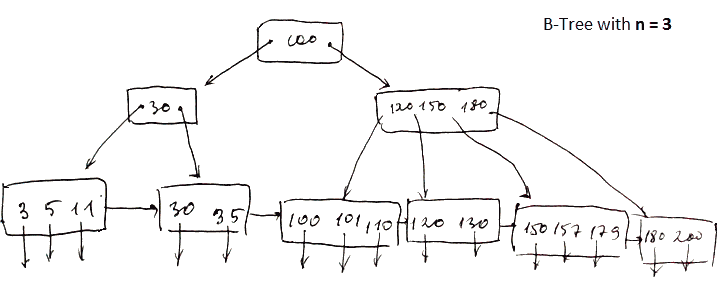• consists of leaf nodes and intermediate nodes

### Order of Tree

parameter $n$ is the order of a tree

• it determines the layout of the block
• each block will have
• $n$ search keys
• $n + 1$ pointer

Example

• suppose our block has size 4096 bytes
• integers are 4 bytes long, pointers are 8 bytes long
• then we want such $n$ that $4n + 8(n + 1) \leqslant 4096$
• that $n = 340$

Example 2

• block size 4096
• 8 bytes per pointer and 8 bytes per key
• $(n + 1) \cdot 8 + n \cdot 8 \leqslant 4096$
• $n = 255$: we can store 256 pointers and 255 keys in one block

### Height

How to estimate height?

• Suppose $128 \times 10^6$ tuples in $R$
• we have a b-tree index with order $n = 255$ on attribute $C$
• assuming all leaf blocks are full, what's the height?
• so there are $\left\lceil \cfrac{128 \times 10^6}{255} \right\rceil$ leaf blocks
• $\left\lceil \cfrac{128 \times 10^6}{255^2} \right\rceil$ blocks at level 2
• $\left\lceil \cfrac{128 \times 10^6}{255^3} \right\rceil$ blocks at level 3
• ...
• $\left\lceil \cfrac{128 \times 10^6}{255^h} \right\rceil$ blocks at level $h$
• so it's logarithm
• $h = \lceil \log_{255} (128 \cdot 10^6) \rceil = 4$
• so #Lookup is 4 + 1 I/Os

What if blocks are half-full?

• since leaves are half-full, they keep 128 records
• $h = \lceil \log_{128} (128 \cdot 10^6) \rceil = 4$
• again 4 + 1 I/Os

### Non-Leaf Node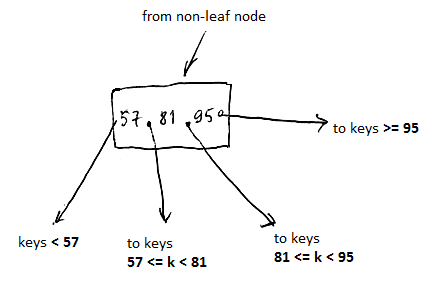• $n$ search keys, $n + 1$ pointers
• first pointer points to keys that are strictly less than the first key
• last pointer points to keys that are greater or equal to the last key
• for in-between pointers, if $k_i$ is a key, and $p_{i-1}$ and $p_i$ are pointers around it, then $p_{i-1} \leqslant k_i < p_i$

### Leaf Node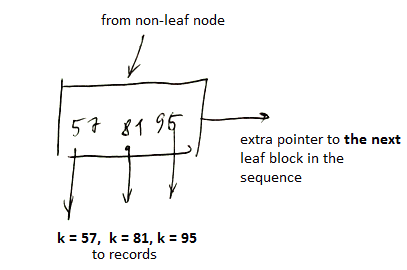• $n$ keys,
• $n$ pointers to actual records, 1 extra pointer to the next leaf in the sequence

## Balancing

Reasons

• I/O cost of looking up is the longest path from the root to a leaf
• so we want our tree be balanced:
• to have paths as short as possible - with all the leaves at the same depth

Idea

• Recall that for $n$ we have $n + 1$ pointers and $n$ keys
• we don't want to have too empty nodes
• so we will require all nodes to be at least half-full

### Size Invariants

nodes are half-full:

• at least $\left\lceil \cfrac{n + 1}{2} \right\rceil$ pointers for non leaf
• at least $\left\lfloor \cfrac{n + 1}{2} \right\rfloor$ pointers for leaf
• the only exception is root: it can contain any number of pointers

for example

•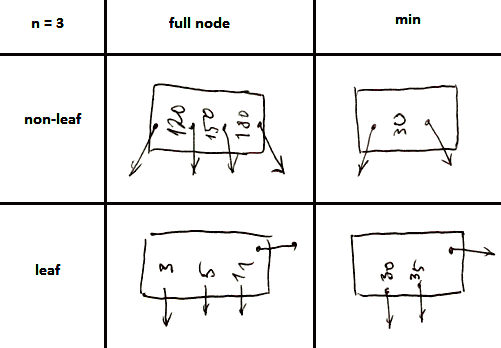• note that for leaf nodes a pointer to the next node counts as well
max # pointers max # keys max # pointers to data min # keys
non-leaf $n + 1$ $n$ $\left\lceil \cfrac{n + 1}{2} \right\rceil$ $\left\lceil \cfrac{n + 1}{2} \right\rceil - 1$
leaf $n + 1$ $n$ $\left\lfloor \cfrac{n + 1}{2} \right\rfloor$ $\left\lfloor \cfrac{n + 1}{2} \right\rfloor$
root $n + 1$ $n$ 2 1

## Operations

Cost of operations are expressed in I/O Model of Computation

### Lookup

suppose we are looking for $k = 35$

•Algorithm

• start at the root
• follow the suitable pointer (as described in #Leaf Node) - this is the left root pointer
• for the next, take the last ($k \geqslant 35$)
• and finally read the block
•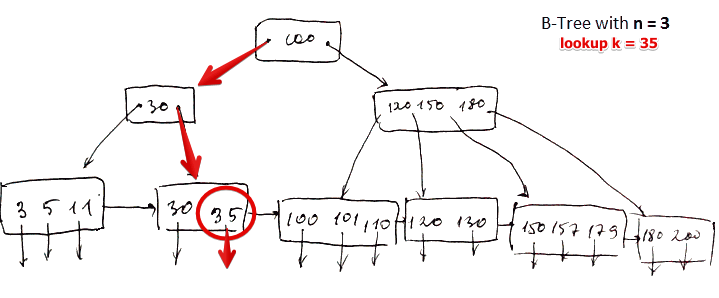#### I/O Cost

• for $k = 35$: 3 I/O
• for $k = 40$ (which doesn't exist): same path as for $k = 35$, cost is 3 I/O
• so I/O cost = the longest path from the root to leaf (which is why we want it balanced)

#### Range Lookups

• BTree supports range queries as well
• such as $35 \leqslant k \leqslant 40$
• we lookup the key corresponding to the left range boundary
• since we have a pointer to the next block, we follow it until we hit the right range boundary
• I/O cost in this case is
• length of the path from the root to the leaf
• then the number of leaves that we need to follow
• and also we need to follow a pointer for each key in the range

### Insert

4 cases

• simple case - space available in leaf
• leaf overflow
• intermediate node overflow
• new root

#### Simple Case

•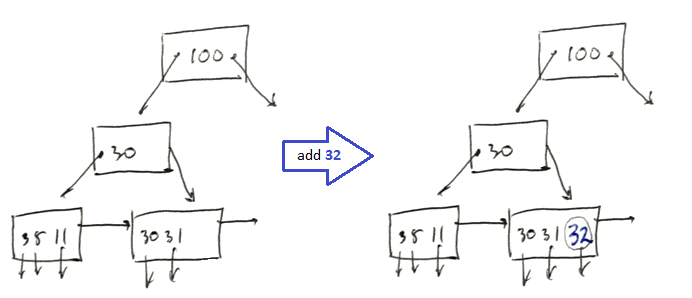• add $k = 32$
• look up $k$ to identify the block where it should be stored
• we have some room there - so just add the record there

#### Leaf Overflow

•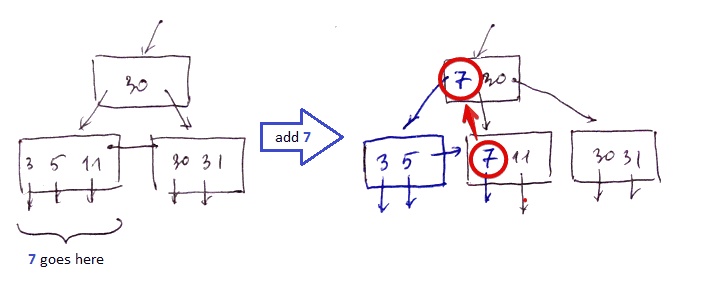• add $k = 7$
• look up the block for 7, but it's fyll
• so we split this block
• create a new one and re-distribute items between them
• this way they both become half-full (the invariant is maintained)
• then in the new block we have some space (Simple Case) and we can put this record there

#### Intermediate Node Overflow

•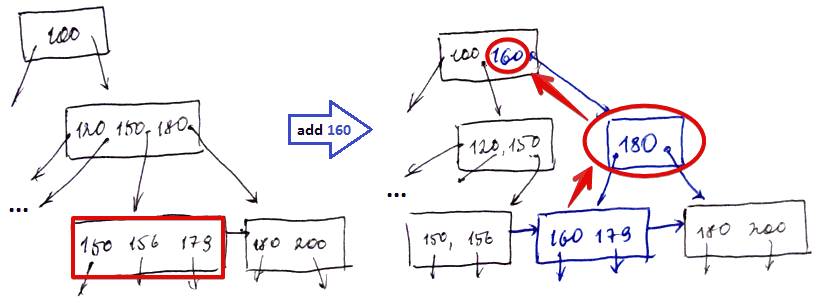• add $k = 160$ ($n = 3$)
• there's no room in $\fbox{150, 156, 179}$ to add $160$ (Leaf Overflow case)
• so we create a new block and re-distribute the keys between them
• since we created a new block, we need to add a pointer to it, but there is no room in $\fbox{120, 150, 180}$
• so we split the intermediate block and move $180$ to the new block
• also need to modify the root block to add pointer to the new block $\fbox{180}$

#### New Root

• sometimes we need to create a new root
•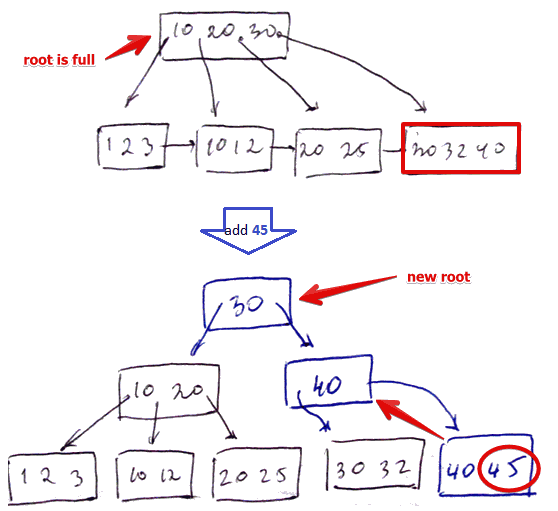• add $k = 45$ ($n = 3$)
• we cannot add it to $\fbox{30, 32, 40}$ (Leaf Overflow case)
• split it to 2 nodes, need to add the pointer to the new node
• cannot add it to $\fbox{10, 20, 30}$ (Intermediate Node Overflow case)
• split it into 2 nodes, need to add the pointer to new block
• but $\fbox{10, 20, 30}$ is a root!
• need to split it to two nodes
• and promote $\fbox{30}$ to the root

#### I/O Cost

• operations
• search
• create a new block (split, write two blocks): 2 operations
• go level up
• so in the worst case 2 I/O at each level + possibly writing a new root (1 I/O)
• then the total cost is
• $\text{depth} + 2 \times \text{depth} + 1 = 3 \times \text{depth} + 1$

### Deleting

Again 4 cases

• Simple - just delete it
• Coalesce Leaf With Siblings
• Redistribute Keys
• Intermediate Nodes Coalescing

#### Coalesce Leaf With Siblings

•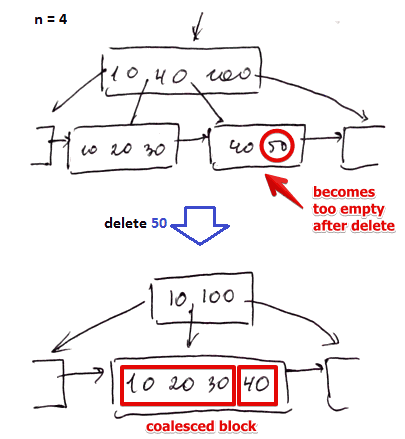• we delete $k = 50$ ($n = 4$)
• we locate the block with 50 and remove this record from it
• now the block becomes too empty (recall #Size Invariants)
• so we coalesce it with $\fbox{10, 20, 30}$
• now we have a new block, and the old one is not needed anymore - we remove it
• additional bookkeeping: need to make sure the next pointer point to the record the old block pointed to

#### Redistribute Keys

•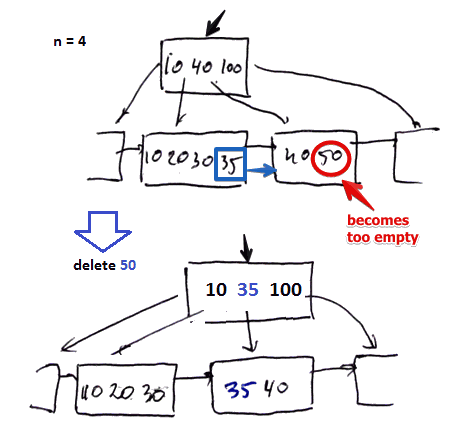• we delete $k = 50$ ($n = 4$)
• when we delete 50, the block that contained it becomes almost empty
• cannot coalesce with $\fbox{10, 20, 30, 35}$ because it's full
• so to fix that we borrow a key from $\fbox{10, 20, 30, 35}$ to become half-full again
• and update the parents accordingly

#### Intermediate Nodes Coalescing

•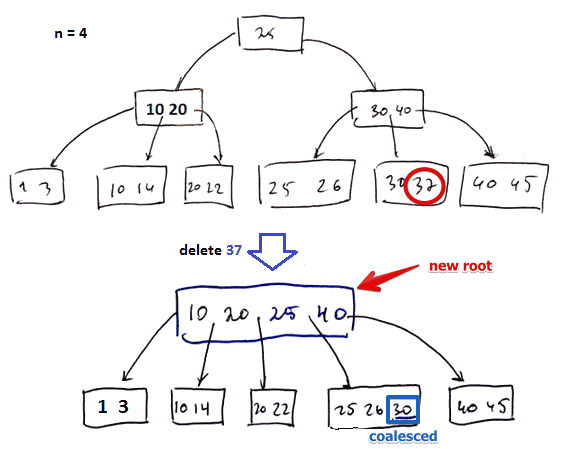• we delete $k = 37$ ($n = 4$)
• first we locate the block with 37 and remove the record from it (Coalesce Leaf With Sibling case)
• since this block $\fbox{30}$ becomes too small we coalesce it with $\fbox{25, 26}$
• need to remove the pointer to the deleted block from the parental node $\fbox{30, 40}$
• i.e. we remove 30 from there
• but then the parental also node becomes too small, so we coalesce it with its sibling as well
• finally we don't need the root $\fbox{25}$ anymore since new block $\fbox{10, 20, 25, 40}$ can reference all the records

#### I/O Cost

Cost

• search: depth of the tree
• remove and regroup: 2 I/Os at each level
• no need to follow the pointer (i.e. don't do +1 as with #Insertion)
• total: $\text{depth} + 2 \times \text{depth} = 3 \times \text{depth}$

## Multiple Keys

### Lexicographical Order

For B-Trees have to be ordered somehow

• we may need to compare tuples in lexicographical order
• so we define this ordering as
• $(x, y, z) \leqslant (x', y', z') \iff \\ x < x' \lor (x = x' \land y < y') \lor (x = x' \land y = y' \land z \leqslant z')$

### Problem with Lexicographical Order

Assume index of (age, salary) pairs with lexicographical order

• btree-lex-ord.png
• query age < 20 - ok
• start at the beginning of index ans scan till see 20
• query salary < 30 - not fine
• linear scan, need to scan everything
• query age < 20 $\land$ sal < 20
• also scan index till see 20,
• meanwhile filtering records with sal < 20
• so using lexicographical ordering doesn't allow all queries we want

need other types of indexes - Multi-Dimensional Indexes

• R-Tree in particular: it's generalization of B-Trees to multidimensional data